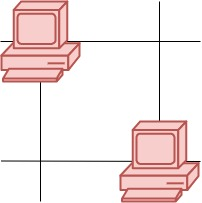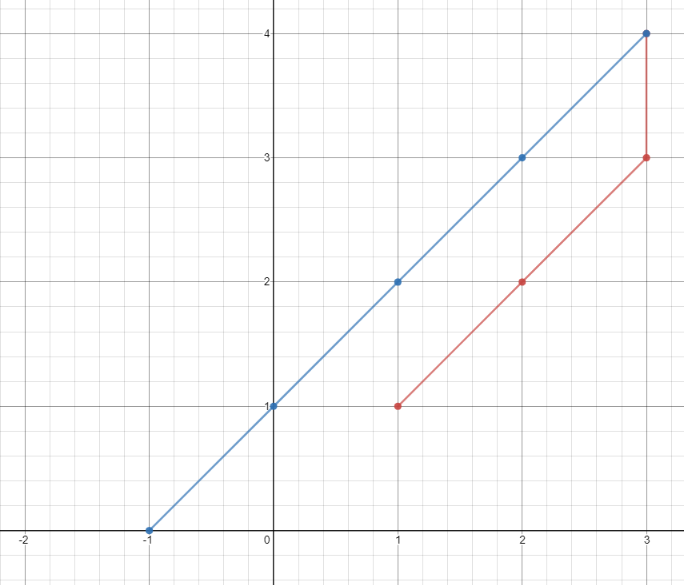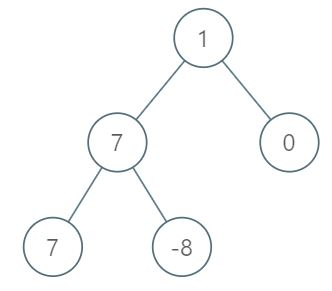## 1267. Count Servers that Communicate

You are given a map of a server center, represented as a `m * n` integer matrix `grid`, where 1 means that on that cell there is a server and 0 means that it is no server. Two servers are said to communicate if they are on the same row or on the same column.

Return the number of servers that communicate with any other server.

Example 1:Input:

``` grid = [[1,0],[0,1]]
```

Output:

``` 0
Explanation: No servers can communicate with others.```

Example 2:Input:

``` grid = [[1,0],[1,1]]
```

Output:

``` 3
Explanation: All three servers can communicate with at least one other server.
```

Example 3:Input:

``` grid = [[1,1,0,0],[0,0,1,0],[0,0,1,0],[0,0,0,1]]
```

Output:

``` 4
Explanation: The two servers in the first row can communicate with each other. The two servers in the third column can communicate with each other. The server at right bottom corner can't communicate with any other server.
```

Constraints:

• `m == grid.length`
• `n == grid[i].length`
• `1 <= m <= 250`
• `1 <= n <= 250`
• `grid[i][j] == 0 or 1`
```class Solution {
public int countServers(int[][] grid) {
int result = 0 ;
int[] a = new int[grid.length];
int[] b = new int[grid.length];

for (int i = 0; i < grid.length; i ++){
for (int j = 0; j < grid.length; j ++){
if (grid[i][j] == 1){
a[i] ++;
b[j] ++;
result ++;
}
}
}

for (int i = 0; i < grid.length; i ++){
for (int j = 0; j < grid.length; j ++){
if (a[i] == 1 && b[j] == 1 && grid[i][j] == 1){
result --;
}
}
}

return result;
}

}```

## 1266. Minimum Time Visiting All Points

On a plane there are `n` points with integer coordinates `points[i] = [xi, yi]`. Your task is to find the minimum time in seconds to visit all points.

You can move according to the next rules:

• In one second always you can either move vertically, horizontally by one unit or diagonally (it means to move one unit vertically and one unit horizontally in one second).
• You have to visit the points in the same order as they appear in the array.

Example 1:Input:

``` points = [[1,1],[3,4],[-1,0]]
```

Output:

``` 7
```

Explanation:

`One optimal path is`

[1,1]

` -> [2,2] -> [3,3] ->`

[3,4]

`-> [2,3] -> [1,2] -> [0,1] ->`

[-1,0]

```
Time from [1,1] to [3,4] = 3 seconds
Time from [3,4] to [-1,0] = 4 seconds
Total time = 7 seconds```

Example 2:

Input:

``` points = [[3,2],[-2,2]]
```

Output:

``` 5
```

Constraints:

• `points.length == n`
• `1 <= n <= 100`
• `points[i].length == 2`
• `-1000 <= points[i], points[i] <= 1000`

```class Solution {
public int minTimeToVisitAllPoints(int[][] points) {
int result = 0;
int prev_x = points;
int prev_y = points;
for (int i = 1; i < points.length; i ++){
int x = points[i];
int y = points[i];

int diff_x = Math.abs(x - prev_x);
int diff_y = Math.abs(y - prev_y);

result += Math.min(diff_x,diff_y)+Math.abs(diff_x-diff_y);

prev_x = x;
prev_y = y;
}
return result;
}
}```

## 1161. Maximum Level Sum of a Binary Tree

Given the `root` of a binary tree, the level of its root is `1`, the level of its children is `2`, and so on.

Return the smallest level `X` such that the sum of all the values of nodes at level `X` is maximal.

Example 1:Input:

```[1,7,0,7,-8,null,null]
```

Output:

```2
```

Explanation:

```Level 1 sum = 1.
Level 2 sum = 7 + 0 = 7.
Level 3 sum = 7 + -8 = -1.
So we return the level with the maximum sum which is level 2.
```

Note:

1. The number of nodes in the given tree is between `1` and `10^4`.
2. `-10^5 <= node.val <= 10^5`

I’m using level order traversal (BFS) here.

```/**
* Definition for a binary tree node.
* public class TreeNode {
*     int val;
*     TreeNode left;
*     TreeNode right;
*     TreeNode(int x) { val = x; }
* }
*/
class Solution {
public int maxLevelSum(TreeNode root) {
int max = 0;
int lv = 1;

int max_lv = 0;

while (! q.isEmpty()){

int size = q.size();
int level_sum = 0;
for (int i = 0; i < size; i ++){
TreeNode cur = q.poll();
if(cur.left != null)
if (cur.right != null)
level_sum += cur.val;
}
if (level_sum > max){
max = level_sum;
max_lv = lv;
}
lv ++;

}
return max_lv;
}

}```

In a row of trees, the `i`-th tree produces fruit with type `tree[i]`.

You start at any tree of your choice, then repeatedly perform the following steps:

2. Move to the next tree to the right of the current tree.  If there is no tree to the right, stop.

Note that you do not have any choice after the initial choice of starting tree: you must perform step 1, then step 2, then back to step 1, then step 2, and so on until you stop.

You have two baskets, and each basket can carry any quantity of fruit, but you want each basket to only carry one type of fruit each.

What is the total amount of fruit you can collect with this procedure?

Example 1:

Input:

```[1,2,1]
```

Output:

```3
```

Explanation:

```We can collect [1,2,1].
```

Example 2:

Input:

```[0,1,2,2]
```

Output:

```3
```

Explanation:

```We can collect [1,2,2].
If we started at the first tree, we would only collect [0, 1].
```

Example 3:

Input:

```[1,2,3,2,2]
```

Output:

```4
```

Explanation:

```We can collect [2,3,2,2].
If we started at the first tree, we would only collect [1, 2].
```

Example 4:

Input:

```[3,3,3,1,2,1,1,2,3,3,4]
```

Output:

```5
```

Explanation:

```We can collect [1,2,1,1,2].
If we started at the first tree or the eighth tree, we would only collect 4 fruits.
```

Note:

1. `1 <= tree.length <= 40000`
2. `0 <= tree[i] < tree.length`

#### First Draft.

Code is accepted, but the quality is a JOKE.

```class Solution {
public int totalFruit(int[] tree) {

if (tree.length <= 2){
return tree.length;
}

int max = 2;
int cur_max = 2;

int a = tree;
int b = tree;

int a_last_index = 0;
Integer b_last_index = null;

boolean a_is_eldest = true;

// get the second <Unique> number
for(int i = 1; i < tree.length; i ++){
if (tree[i] != a){
b = tree[i];
b_last_index = i;
break;
} else {
// And we have to update last index of a as well
cur_max ++;
a_last_index = i;
a_is_eldest = false;
}
}
// update max here
max = Math.max(max, cur_max);

// if only single unique number in the array...
if (b_last_index == null){
return tree.length;
}

// Scan from the right of the second unique number
for(int i = b_last_index+1; i < tree.length; i ++){
int cur = tree[i];

if(cur == a){

cur_max ++;
a_last_index = i;
a_is_eldest = false;

} else if (cur == b){

cur_max ++;
b_last_index = i;
a_is_eldest = true;

} else {

// Third unique number appeared!
// Remove eldest, and reduce current max to the last occurance of the number to be removed.
if(a_is_eldest){
cur_max = i - a_last_index;
a = cur;
a_last_index = i;
a_is_eldest = false;
} else {
cur_max = i - b_last_index;
b = cur;
b_last_index = i;
a_is_eldest = true;
}
}
max = Math.max(max, cur_max);
}
return max;
}
}```

#### Code after refactoring:

```class Solution {
public int totalFruit(int[] tree) {

if (tree.length <= 2){
return tree.length;
}

int max = 2;
int cur_max = 1;

int a = tree;
int a_last_index = 0;

Integer b = null;
Integer b_last_index = null;

boolean a_is_eldest = true;

for(int i = 1; i < tree.length; i ++){
int cur = tree[i];

if(cur == a){
cur_max ++;
a_last_index = i;
a_is_eldest = false;

} else if (b == null || cur == b) {

if(b == null){
b = cur;
}
cur_max ++;
b_last_index = i;
a_is_eldest = true;

} else {

if(a_is_eldest){
cur_max = i - a_last_index;
a = cur;
a_last_index = i;
a_is_eldest = false;
} else {
cur_max = i - b_last_index;
b = cur;
b_last_index = i;
a_is_eldest = true;
}

}
max = Math.max(max, cur_max);
}
return max;
}
}```

There could be more things for refactoring, putting that aside, can we use any data structure?

Maybe LinkedHashMap<Type, LastSeenIndex> in access order mode?  it’s an overkill for ‘collecting two types’,  but could be useful for 3 or more types.

Starting by copy & paste from LRUCache. I added a variable to save the value of the most recent removed entry. The value of it is updated before the eldest entry is removed.

#### The modified LRUCache

```class LRUCache extends LinkedHashMap<Integer, Integer>{
private int capacity;

// Save the latest index of the recent removed item.
int index_removed = 0;

public LRUCache(int capacity) {
super(capacity, 0.75F, true);
this.capacity = capacity;
}

public int get(int key) {
return super.getOrDefault(key, -1);
}

public void put(int key, int value) {
super.put(key, value);
}

@Override
protected boolean removeEldestEntry(Map.Entry<Integer, Integer> eldest) {

// If the eldest has to be removed, save it's value (most recent index)
if(size() > capacity){
index_removed = eldest.getValue();
}

return size() > capacity;
}
}```

Then, update the main function… and combine them

```class Solution {

public static final int TYPES_ALLOWED = 2;

public int totalFruit(int[] tree) {

if (tree.length <= TYPES_ALLOWED){
return tree.length;
}

int cur_max = 1;
int max = 1;

// For a follow-up of more than 2 types is allowed.. just change the number here
LRUCache map = new LRUCache(TYPES_ALLOWED);

map.put(tree, 0);

for(int i = 1; i < tree.length; i ++){
int cur = tree[i];
if (map.get(cur) != -1 || map.size() < 2){
cur_max ++;
map.put(cur, i);
} else {
map.put(cur, i);
cur_max = i - map.index_removed;
}

max = Math.max(max, cur_max);

}
return max;
}
}

private int capacity;
int index_removed = 0;

public LRUCache(int capacity) {
super(capacity, 0.75F, true);
this.capacity = capacity;
}

public int get(int key) {
return super.getOrDefault(key, -1);
}

public void put(int key, int value) {
super.put(key, value);
}

@Override
protected boolean removeEldestEntry(Map.Entry<Integer, Integer> eldest) {
if(size() > capacity){
index_removed = eldest.getValue();
}
return size() > capacity;
}
}```

Time complexity. O(n). basically going through all elements once (n),  times LRUCache O(1).

This is basically a sliding window solution, while the Map is used for maintaining the valid window status.

Well, we can also use other Collections, giving the accepted types is two. The search and insertion time have to be counted after the number of accepted types is increased. So a Hash* will have a better performance.

Can we use a LinkedHashSet? Yes, then giving the type to be removed, find the closest index before the current index.

## 973. K Closest Points to Origin

We have a list of `points` on the plane.  Find the `K` closest points to the origin `(0, 0)`.

(Here, the distance between two points on a plane is the Euclidean distance.)

You may return the answer in any order.  The answer is guaranteed to be unique (except for the order that it is in.)

Example 1:

Input:

```points = [[1,3],[-2,2]], K = 1
```

Output:

```[[-2,2]]
```

Explanation:

```The distance between (1, 3) and the origin is sqrt(10).
The distance between (-2, 2) and the origin is sqrt(8).
Since sqrt(8) < sqrt(10), (-2, 2) is closer to the origin.
We only want the closest K = 1 points from the origin, so the answer is just [[-2,2]].
```

Example 2:

Input:

```points = [[3,3],[5,-1],[-2,4]], K = 2
```

Output:

```[[3,3],[-2,4]]
(The answer [[-2,4],[3,3]] would also be accepted.)
```

Note:

1. `1 <= K <= points.length <= 10000`
2. `-10000 < points[i] < 10000`
3. `-10000 < points[i] < 10000`
A acceptable but definitely no the best solution….
In PHP:
1. a ** b means power (a,b)
2.  <=>, the spaceship operator,  used by ‘comparator’
1. if left < right return -1
2. if left > right return 1
3. if left == right return 0
```class Solution {

/**
* @param Integer[][] \$points
* @param Integer \$K
* @return Integer[][]
*/
function kClosest(\$points, \$K) {
usort(\$points, function (\$a, \$b){
return (\$a**2 + \$a**2) <=> (\$b**2 + \$b**2);
});

\$result = [];
\$i = 0;
foreach(\$points as \$p){
if(\$i >= \$K){
break;
}
\$result[] = \$p;
\$i ++;

}
return \$result;
}
}```

## 102. Binary Tree Level Order Traversal

Given a binary tree, return the level order traversal of its nodes’ values. (ie, from left to right, level by level).

For example:
Given binary tree `[3,9,20,null,null,15,7]`,

```    3
/ \
9  20
/  \
15   7
```

return its level order traversal as:

```[
,
[9,20],
[15,7]
]

Straight forward solution:
root node in => left and right children out . -- Lv1
lv 1 nodes in => lv 2 nodes out......
until no more next lv.```
```/**
* Definition for a binary tree node.
* class TreeNode {
*     public \$val = null;
*     public \$left = null;
*     public \$right = null;
*     function __construct(\$value) { \$this->val = \$value; }
* }
*/
class Solution {

/**
* @param TreeNode \$root
* @return Integer[][]
*/
function levelOrder(\$root) {
\$q = [];
\$result = [];

if(\$root === null){
return \$result;
}
\$q[] = \$root;
while (count(\$q) > 0){
\$current_level = [];
\$current_result = [];
foreach (\$q as \$node){
if (\$node->left !== null)
\$current_level[] = \$node->left;

\$current_result[] = \$node->val;

if (\$node->right !== null)
\$current_level[] = \$node->right;
}
\$q = \$current_level;
\$result [] = \$current_result;
}

return \$result;
}
}```
```/**
* Definition for a binary tree node.
* public class TreeNode {
*     int val;
*     TreeNode left;
*     TreeNode right;
*     TreeNode(int x) { val = x; }
* }
*/
class Solution {
public List<List<Integer>> levelOrder(TreeNode root) {
List<List<Integer>> r = new ArrayList<>();

if(root == null){
return r;
}

while(!q.isEmpty()){

int sizeSnapshot = q.size();
List<Integer> current_level = new ArrayList<>();

for(int i = 0; i < sizeSnapshot; i ++){
TreeNode t = q.poll();
if(t.left != null){
}

if(t.right != null){
}
}
}

return r;
}
}```

## 189. Rotate Array

Given an array, rotate the array to the right by k steps, where k is non-negative.

Example 1:

Input:

``[1,2,3,4,5,6,7]` and`

k

` = 3`

Output:

``[5,6,7,1,2,3,4]``

Explanation:

```rotate 1 steps to the right: `[7,1,2,3,4,5,6]` rotate 2 steps to the right: ```[6,7,1,2,3,4,5]
```rotate 3 steps to the right: `[5,6,7,1,2,3,4]````

Example 2:

Input:

``[-1,-100,3,99]` and`

k

` = 2`

Output:

` [3,99,-1,-100]`

Explanation:

` rotate 1 steps to the right: [99,-1,-100,3] rotate 2 steps to the right: [3,99,-1,-100]`

Note:

• Try to come up as many solutions as you can, there are at least 3 different ways to solve this problem.
• Could you do it in-place with O(1) extra space?

In place rotate

```class Solution {

/**
* @param Integer[] \$nums
* @param Integer \$k
* @return NULL
*/
function rotate(&\$nums, \$k) {
\$c = count(\$nums);
\$steps = 0;
\$start = 0;

while(\$steps < \$c){
\$i = \$start;
\$prev = \$nums[\$i];
do{
\$next = (\$i+\$k)%\$c;
\$temp = \$nums[\$next];
\$nums[\$next] = \$prev;
\$prev = \$temp;
\$i += \$k;
\$i = \$i % \$c;
\$steps++;
}while(\$start != \$i);
\$start ++;

}

}
}```

## 406. Queue Reconstruction by Height

Suppose you have a random list of people standing in a queue. Each person is described by a pair of integers `(h, k)`, where `h` is the height of the person and `k` is the number of people in front of this person who have a height greater than or equal to `h`. Write an algorithm to reconstruct the queue.

Note:
The number of people is less than 1,100.

Input: [[7,0], [4,4], [7,1], [5,0], [6,1], [5,2]] Output: [[5,0], [7,0], [5,2], [6,1], [4,4], [7,1]]

Example

Solution:

```<?php

class Solution {

/**
* @param Integer[][] \$people
* @return Integer[][]
*/
function reconstructQueue(\$people) {
usort(\$people, function(\$p1, \$p2){
return (\$p1 !== \$p2)?
\$p2 <=> \$p1 : \$p1 <=> \$p2;
});
\$result = [];
foreach(\$people as \$p){
array_splice(\$result, \$p, 0, [\$p]);
}

return \$result;
}
}

?>```

[[7,0], [4,4], [7,1], [5,0], [6,1], [5,2]]

The higher the person is, the more importance it has for other persons

• So starting sort by height, DESC
• Then for each height level, sort by # of higher heads in the front, ASC
• (Just like ‘ORDER BY h DESC, k ASC’ in SQL)

Now we have:

More Important to Others <===> Less Important to Others

[7,0]    [7,1]     [6,1]    [5,0]    [5,2]   [4,4]

Then set group height = 7 to the result

[7,0] [7,1]  ;   TBD: [6,1]    [5,0]    [5,2]   [4,4]

Now set group  height = 6. It could be places any where, since h=7 can’t see them, so the position is determined by their value of k. for example: [6,1]. there’s one higher/equal height person ahead, we can simply insert it right after k is matched, and push everyone else backwards safely since they don’t care about lower person moving around.

```   0     1     2     3     4  ..... (index)

[7,0] [6, 1] [7,1]  ;                TBD:    [5,0]    [5,2]   [4,4]

[5,0] [7,0] [6, 1] [7,1]  ;          TBD:    [5,2]   [4,4]

[5,0] [7,0] [5,2] [6, 1] [7,1]  ;    TBD:    [4,4]

[5,0] [7,0] [5,2] [6, 1] [4,4]  [7,1]```

In PHP, `array_splice(\$result, \$p, 0, [\$p]); ` means insert p to position p[i] and move people behind backwards